﻿ 基于EMD分解的海浪有效波高短期预测研究 Short-Term Prediction of Significant Wave Height Based on EMD Decomposition

Advances in Marine Sciences
Vol. 06  No. 02 ( 2019 ), Article ID: 30628 , 13 pages
10.12677/AMS.2019.62007

Short-Term Prediction of Significant Wave Height Based on EMD Decomposition

Shiyi Kan*, Ting Yu, Li Liu

College of Science, China University of Petroleum (East China), Qingdao Shandong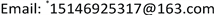Received: May 13th, 2019; accepted: May 28th, 2019; published: Jun. 4th, 2019ABSTRACT

Waves are closely related to human activities at sea, and play an important role in the fields of navigation, marine development, disaster prevention and mitigation. Wave prediction, especially the prediction of significant wave height, has always been the focus of ocean research. Because of the random characteristics and uncertainties of waves, the accuracy of wave prediction is still the key problem to be solved. In this paper, the wave data in Bohai Sea are deeply studied to predict the short-term significant wave height by a hybrid model based on empirical mode decomposition (EMD) and support vector regression (SVR). Empirical mode decomposition (EMD) can adaptively decompose non-stationary time series into a series of intrinsic mode functions (IMFs) and residuals with frequencies from high to low. According to the severity of trend changes of the IMFs, different kernel functions are selected for support vector regression prediction. Then, the weighted combination of each prediction component is used to obtain the accurate prediction value of the original series. The short-term prediction experiment of significant wave height in Bohai Sea in 2012 shows that the hybrid model based on empirical mode decomposition (EMD) and support vector regression model (SVR) is more accurate than the single support vector regression model.

Keywords:Empirical Mode Decomposition, Support Vector Regression, Prediction of Significant Wave Height, Hybrid ModelCopyright © 2019 by author(s) and Hans Publishers Inc.1. 引言

EMD方法是分析非稳态信号的一种独特分析方法，在理论上可以用于任何类型的信号分解，现主要用于地球物理探测、地震工程、结构损害侦测、潜艇设计、血压变化和心律不整、卫星资料分析等各项研究  。EMD方法还未普遍应用于海浪有效波高的预测，本文只是一次尝试。应用EMD方法在海浪数据预测海浪有效波高的实验中有很好的效果，但在用风场数据预测海浪有效波高的实验中面临一个问题：风和浪分解后的本征模函数个数不一样，进行SVR预测时找不到有效参数。这是目前待解决的问题。

2. 研究范围和使用的数据Figure 1. Significant wave height

3. 模型的建立

3.1. 经验模式分解

EMD分解方法是基于以下假设条件：

1) 数据至少有两个极值，一个最大值和一个最小值；

2) 数据的局部时域特性是由极值点间的时间尺度唯一确定；

3) 如果数据没有极值点但有拐点，则可以通过对数据微分一次或多次求得极值，然后再通过积分来获得分解结果。

· 找出原时间数据序列 $X\left(t\right)$ 所有的极大值点和极小值点并用三次样条插值函数拟合形成原数据的上包络线 $u\left(t\right)$ 和下包络线 $I\left(t\right)$ ，取上包络线和下包络线的均值记作 $m\left(t\right)$ ，即 $m\left(t\right)=\left[I\left(t\right)+u\left(t\right)\right]/2$

· 将原数据序列 $X\left(t\right)$ 减去该平均包络 $m\left(t\right)$ ，得到一个新的数据序列 $h\left(t\right)$ ，即 $h\left(t\right)=X\left(t\right)-m\left(t\right)$

· 验证 $h\left(t\right)$ 是否满足IMF的条件，若还存在负的局部极大值和正的局部极小值，则执行步骤1)到步骤2)，直到 $h\left(t\right)$ 是本征模函数为止。

· 经过n个转移过程后，将得到第n个IMF组分 $IM{F}_{n}\left(t\right)=c\left(t\right)$ 和相应的残差，重复整个算法，当残差变为单调函数时，停止迭代。

· 最终得到分解后的时间数据序列方程： $X\left(t\right)=\underset{i=1}{\overset{n}{\sum }}im{f}_{i}\left(t\right)+r\left(t\right)$

3.2. 支持向量回归

3.2.1. 支持向量回归的应用性

3.2.2. 支持向量回归的理论基础

$y\left(h\right)={W}^{\text{T}}•\varphi \left(h\right)+b,\text{\hspace{0.17em}}\text{\hspace{0.17em}}W\in {R}^{m}$ (1)

$R\left(w\right)=\frac{1}{2}{W}^{\text{T}}W+C\underset{i=1}{\overset{N}{\sum }}{J}^{\prime }\left({y}_{i},{d}_{i}\right)$ (2)

${J}^{\prime }\left({y}_{i},{d}_{i}\right)=\left\{\begin{array}{l}|{y}_{i}-{d}_{i}|-\epsilon \text{}|{y}_{i}-{d}_{i}|\ge \epsilon \\ 0\text{}其他\end{array}$ (3)

$\left\{\begin{array}{l}\mathrm{min}J\left(w,\stackrel{\to }{e}\right)=\frac{1}{2}{W}^{\text{T}}W+\frac{C}{2}\underset{i=1}{\overset{N}{\sum }}{e}_{i}^{2}\\ \text{s}\text{.t}\text{\hspace{0.17em}}\text{\hspace{0.17em}}{y}_{i={W}^{\text{T}}}\varphi \left({h}_{i}\right)+b+{e}_{i},i=1,2,\cdots ,N\end{array}$ (4)

$L\left(w,b,e,a\right)=J\left(w,e\right)-\underset{i=1}{\overset{N}{\sum }}{a}_{i}\left({W}^{\text{T}}\varphi \left({h}_{i}\right)+b+{e}_{i}-{y}_{i}\right)$ (5)

$\left\{\begin{array}{l}\frac{\partial L}{\partial {\alpha }_{i}}=0\to W\Phi \left({h}_{i}\right)+b+{e}_{i}-{y}_{i}\\ \frac{\partial L}{\partial b}=0\to \underset{i=1}{\overset{N}{\sum }}{\alpha }_{i}=0\\ \frac{\partial L}{\partial {e}_{i}}=0\to {\alpha }_{i}=C{e}_{i}\\ \frac{\partial L}{\partial W}=0\to W=\underset{i=1}{\overset{N}{\sum }}{\alpha }_{i}\Phi \left({h}_{i}\right)\end{array}$ (6)

$\left[\begin{array}{cc}{Q}_{N}+{C}^{-1}& {e}_{i}\\ {e}_{i}& 0\end{array}\right]\left[\begin{array}{c}{a}_{N}\\ b\end{array}\right]=\left[\begin{array}{c}{y}_{N}\\ 0\end{array}\right]$ (7)

$k\left({h}_{i},{h}_{j}\right)=\mathrm{exp}\left(-{‖{h}_{i}-{h}_{j}‖}_{2}^{2}/{\beta }^{2}\right),\beta >0$ (8)

$K\left(x,{x}_{i}\right)=\mathrm{exp}\left(-\gamma {‖x-{x}_{i}‖}^{2}\right)$ (9)

$y\left(h\right)=\underset{i=1}{\overset{N}{\sum }}{a}_{i}k\left({h}_{i},{h}_{j}\right)+b$ (10)

3.3. 联合EMD与SVR的有效波高短期预测模型建立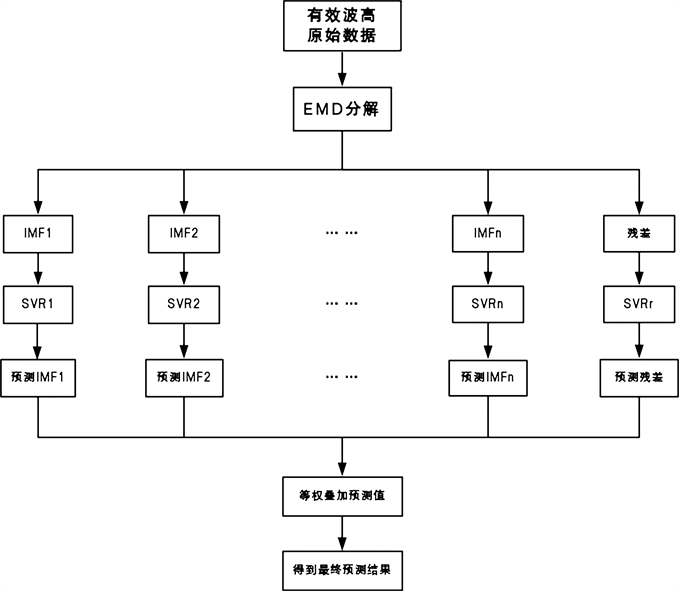Figure 2. EMD-SVR model building diagram

SVR回归算法可实现线性或非线性的函数映射，其训练过程实际就是一个优化计算的过程，故可应用SVR实现组合预测。其基本思想是：将各个IMF的SVR同时刻的预测结果作为输入，将同时刻的相应的实际值作为输出。然后对网络进行足够的学习训练，直到找到最优参数为止  。从而使得各个参加组合预测的分量和实际输出值之间建立一种非线性映射关系。对于训练好的网络，当输入端为各个不同IMF的SVR预测值时，其输出即为组合后的最终预测值。

3.4. 统计参数

$RMSE=\sqrt{\frac{1}{n}\sum {\left({x}_{i}-{y}_{i}\right)}^{2}}$ (11)

$R=\frac{\sum \left({x}_{i}-\stackrel{¯}{x}\right)\left({y}_{i}-\stackrel{¯}{y}\right)}{\sqrt{\sum {\left({x}_{i}-\stackrel{¯}{x}\right)}^{2}\sum {\left({y}_{i}-\stackrel{¯}{y}\right)}^{2}}}$ (12)

$IA=1-\frac{\sum {\left({y}_{i}-{x}_{i}\right)}^{2}}{\sum {\left(|{y}_{i}-\stackrel{¯}{x}|+|{x}_{i}-\stackrel{¯}{x}|\right)}^{2}}$ (13)

4. 渤海海浪有效波高预测实验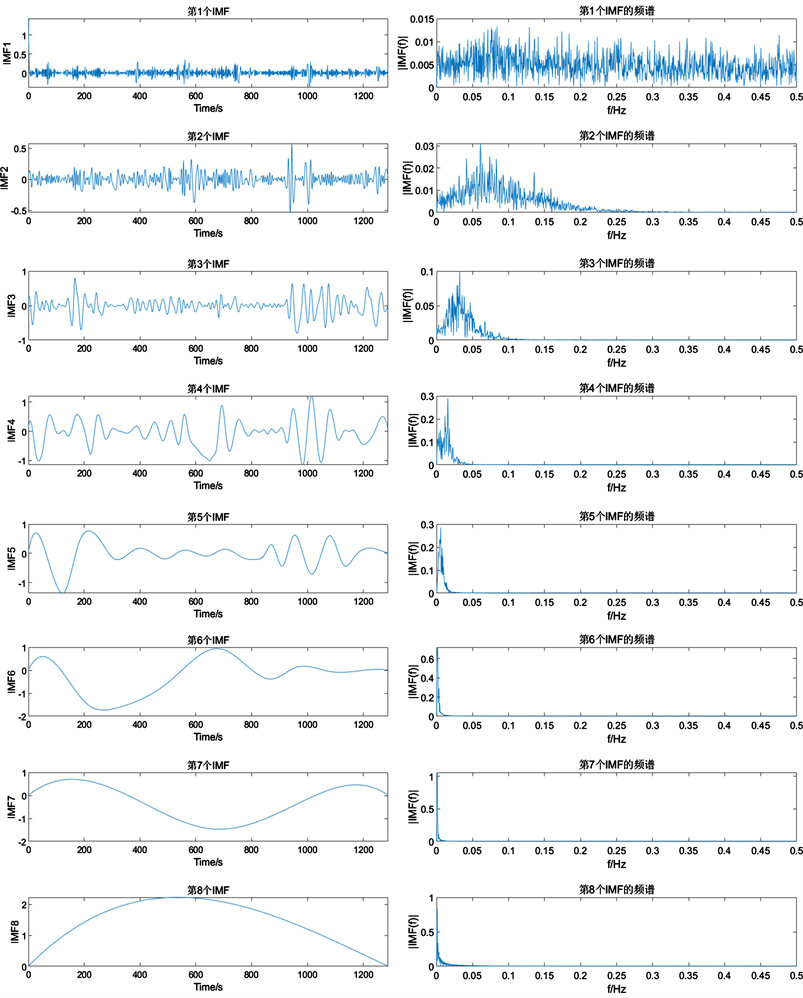Figure 3. EMD decomposition graph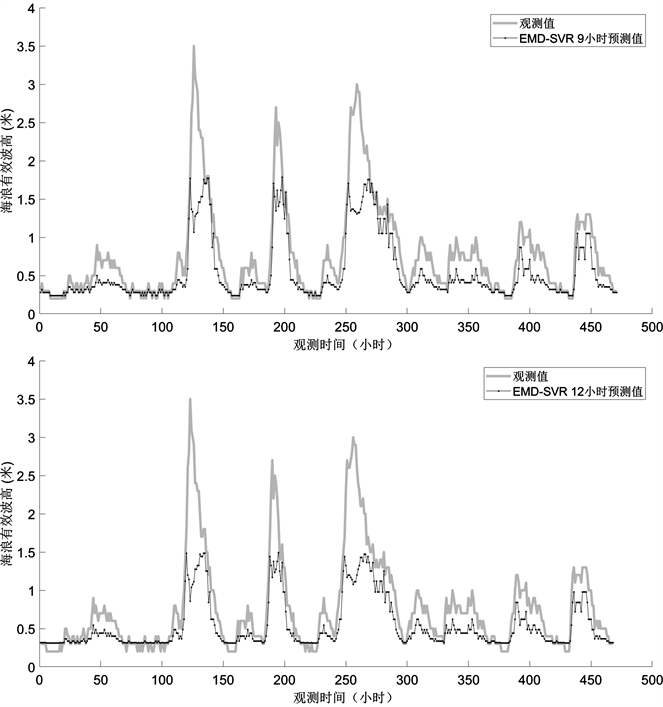Figure 4. Predicted and observed values of EMD-SVR at different time pointsTable 1. Error index of EMD-SVR prediction at different time points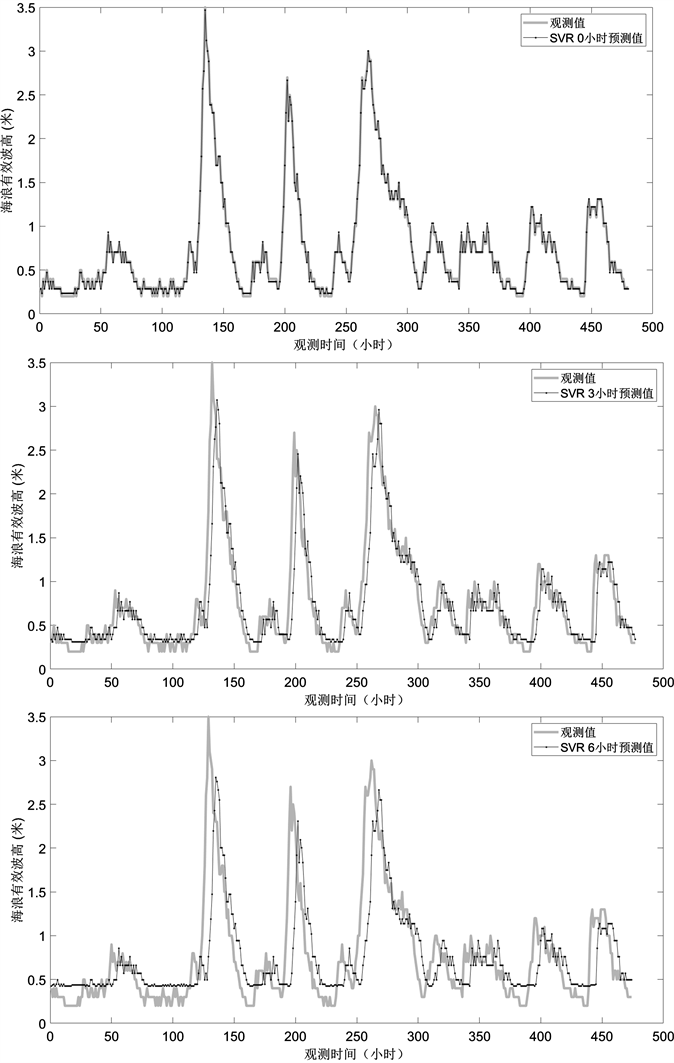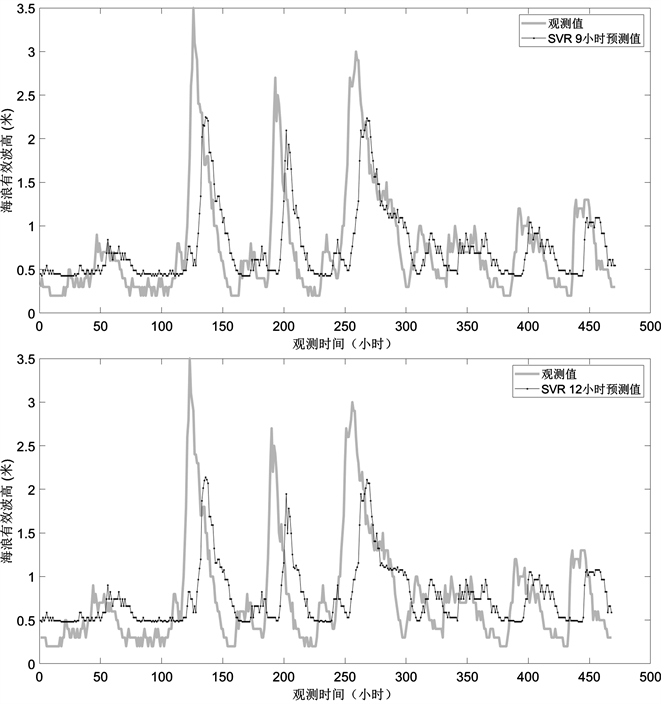Figure 5. Predicted and observed values of SVR at various time pointsTable 2. Error index of SVR prediction at different time points

5. 结论

Short-Term Prediction of Significant Wave Height Based on EMD Decomposition[J]. 海洋科学前沿, 2019, 06(02): 51-63. https://doi.org/10.12677/AMS.2019.62007

1. 1. Duan, W.Y., Han, Y., Huang, L.M., Zhao, B.B. and Wang, M.H. (2016) A Hybrid EMD-SVR Model for the Short-Term Prediction of Significant Wave Height. Ocean Engineering, 124, 54-73. https://doi.org/10.1016/j.oceaneng.2016.05.049

2. 2. Huang, W., Shen, Z., Huang, N.E. and Fung, Y.C. (1998) Engineering Analysis of Biologicall Variables: An Example of Blood Pressure over 1 Day. Proceedings of the National Academy of Sciences of the United States of America, 95, 4816-4821. https://doi.org/10.1073/pnas.95.9.4816

3. 3. Rilling, G., Flandrin, P. and Goncalves, E. (2003) On Empirical Mode Decomposition and Its Algorithms. Proceeding of IEEE EUSIPCO Work, 2003, 8-11.

4. 4. 潘红宇, 编著. 时间序列分析[M]. 北京: 对外经济贸易大学出版社, 2006.

5. 5. Balocchi, R., Menicucci, D. and Varanini, M. (2003) Empirical Mode Decomr Position to an Approach the Problem of Detecting Sources from a Reduced Number of Mixtures. Proceeding of the 25th Annual International Conference of the IEEE EM BS, 4, 2443-2446.

6. 6. 张春友, 闫伟, 吴晓强, 等. 基于SVR的图书馆数字资源服务绩效评价方法[J]. 现代电子技术, 2018, 41(22): 21-24, 29.

7. 7. 李亚巍, 卞双. 支持向量回归机理论及其应用[J]. 中国电力教育, 2005(S2): 183-184.

8. 8. 肖建, 于龙, 白裔峰. 支持向量回归中核函数和超参数选择方法综述[J]. 西南交通大学学报, 2008, 43(3): 297-303.

9. 9. 杨玫, 刘瑜, 孔波. SVR模型参数选择方法的研究[J]. 计算机时代, 2009(11): 53-55.

10. 10. 姚琦, 牛瑞卿, 赵金童, 等. 基于经验模式分解–支持向量机的滑坡位移预测方法研究[J]. 安全与环境工程, 2017, 24(1): 26-32.

11. 11. 王巍, 赵宏, 梁朝晖, 等. 基于EMD和SVR的混合智能预测模型及实证研究[J]. 计算机工程与应用, 2012, 48(4): 225-227.

NOTES

*通讯作者。